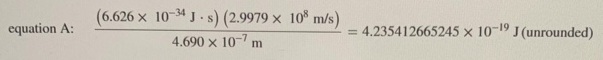# Problem: To how many significant figures should each answer be rounded?After rounding, the answer to equation A should havea. 1 significant figureb. 5 significant figurec. 2 significant figured. 3 significant figuree. 4 significant figure

###### FREE Expert Solution

We are asked for the number of significant figures in the answer to the problem.

The number of significant figures depends on the operation we use:

1.  addition or subtraction - the least number of decimal places will determine the final answer.

2. multiplication or division - the least number of significant figures will determine the final answer.

We operate the ones in the brackets first in this order : () → [] → {}

91% (196 ratings)###### Problem Details

To how many significant figures should each answer be rounded?After rounding, the answer to equation A should have

a. 1 significant figure

b. 5 significant figure

c. 2 significant figure

d. 3 significant figure

e. 4 significant figure

Frequently Asked Questions

What scientific concept do you need to know in order to solve this problem?

Our tutors have indicated that to solve this problem you will need to apply the Significant Figures: In Calculations concept. You can view video lessons to learn Significant Figures: In Calculations. Or if you need more Significant Figures: In Calculations practice, you can also practice Significant Figures: In Calculations practice problems.